## General Chemistry

In the previous post, we discussed the nature of light and how the sunlight or the light from an ordinary light bulb (white light) contains a mixture of almost all the colors in the rainbow. This can be confirmed by passing white light through a prism which produces a rainbow of colors as different wavelengths pass through the prims at different rates: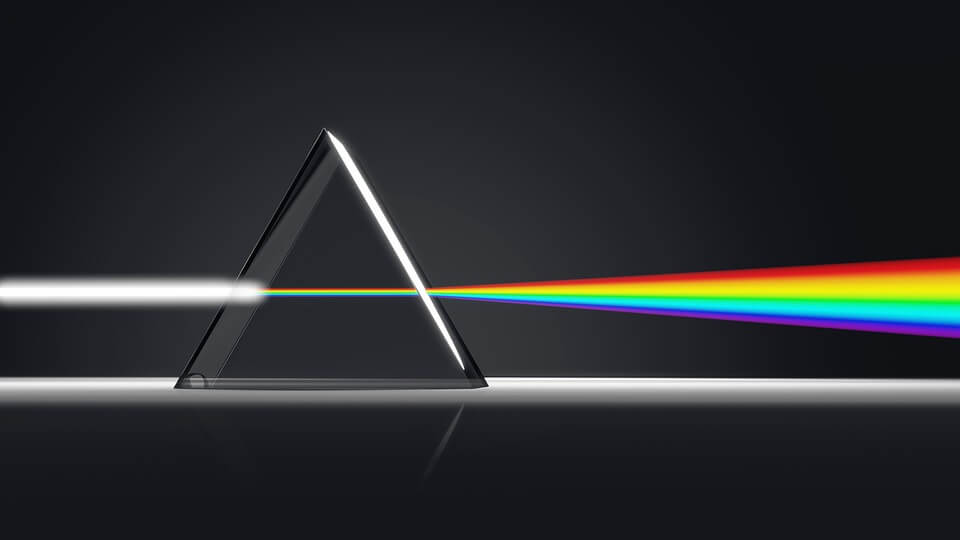Now, what is interesting is that when a discharge tube containing a specific element, such as hydrogen, neon, sodium, is subjected to electric current, white light is not produced but a light of specific color is seen. In the case of hydrogen, it is a pinkish light.To understand why this happens, first, let’s recall how light from an element or a compound is produced. You need to remember that light is an emission of electromagnetic radiation. The emission occurs when an electron(s) in the atom falls from an excited (high energy) to the ground, or in general, a lower energy level: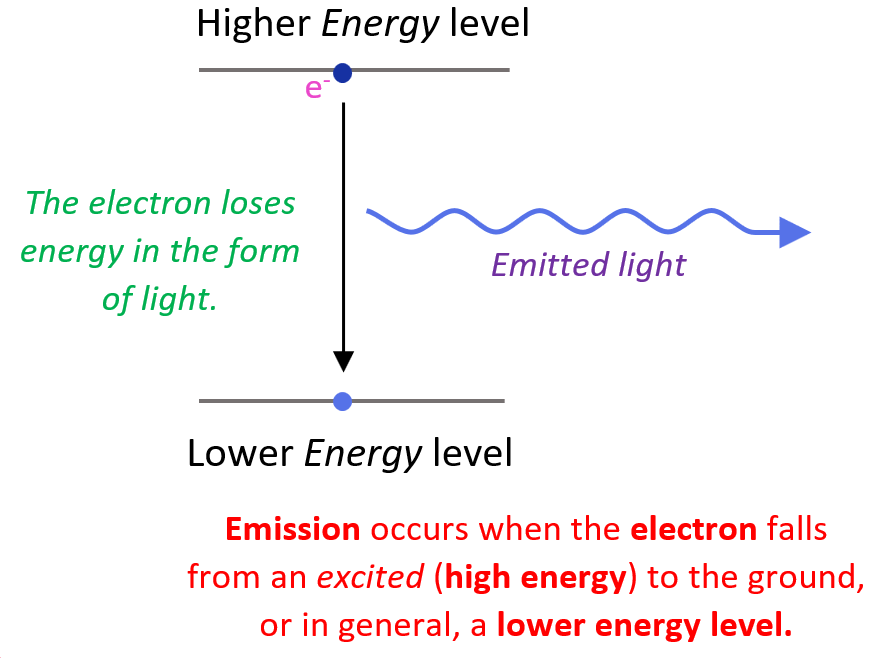Now, you may be wondering how the electron appears at a higher energy level in the first place. This is a result of the electron absorbing energy such as heat, electrical current, electron bombardment and etc.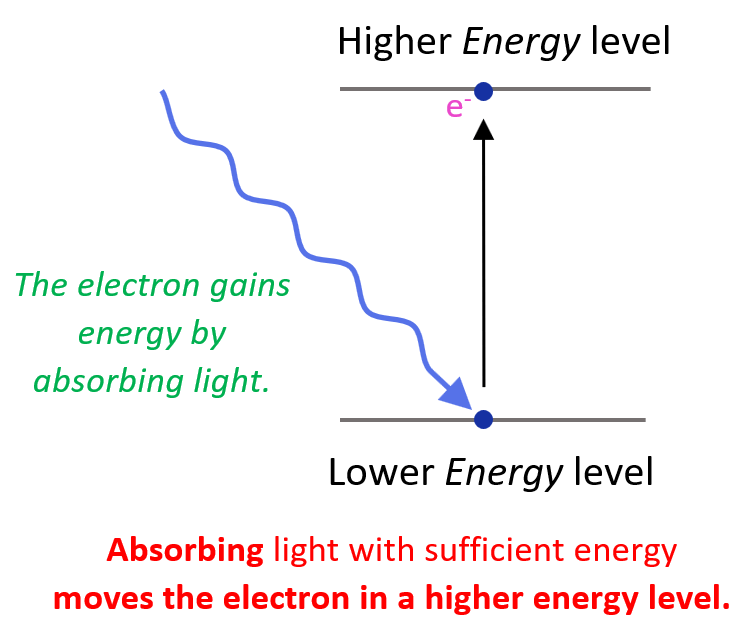So, remember, absorbing light with sufficient energy moves the electron to a higher energy level, and when it falls back to a lower energy level, light is emitted as energy is lost.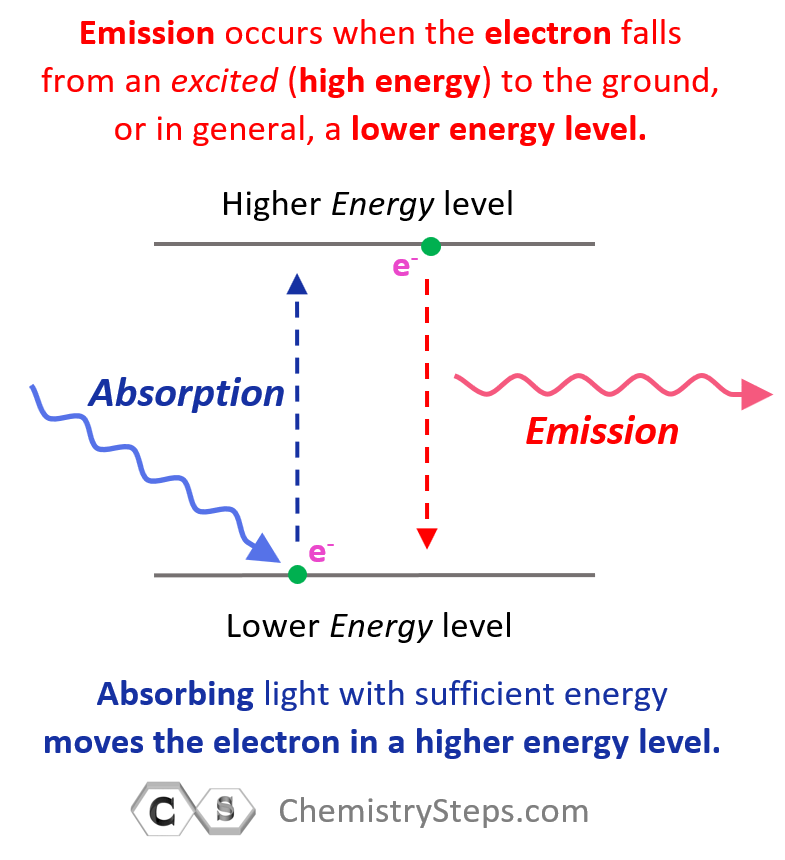Going back to the question of why hydrogen does not emit white light. First, how was it confirmed that only photons of specific wavelengths were emitted? For this, a prism was used which could separate all the wavelengths of the light emitted by hydrogen, and only a few wavelengths rather than a full rainbow of colors were seen.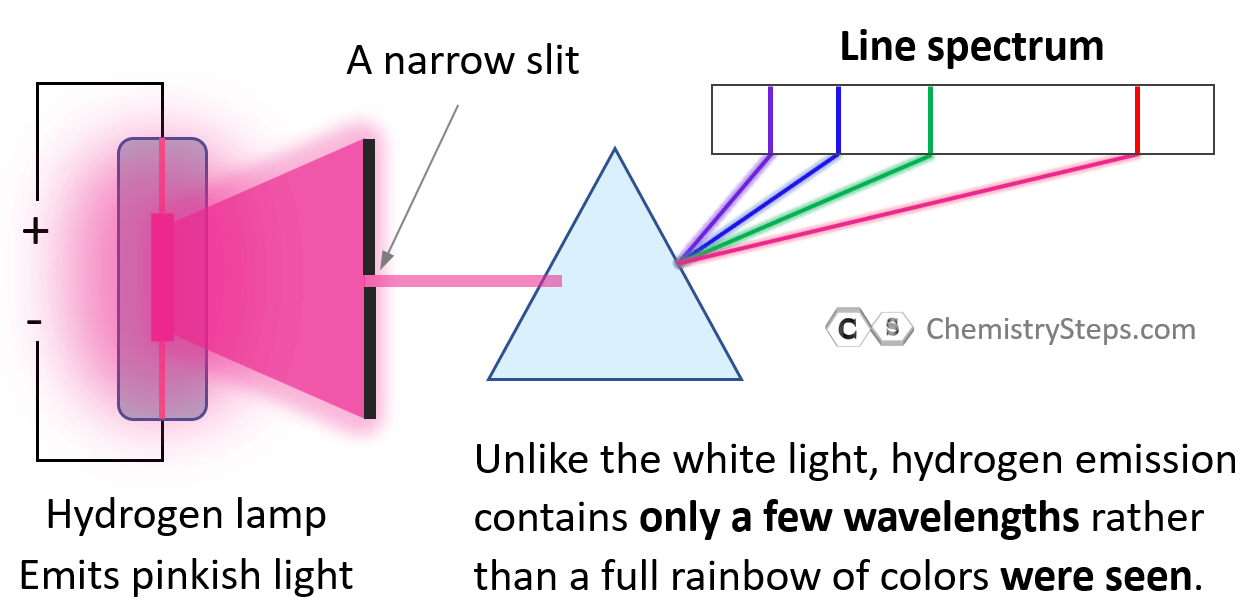This is called a line spectrum and it is unique to each element. This phenomenon was explained by a Danish physicist Niels Bohr (1885–1962), who was at the time working in Rutherford’s lab. Bohr suggested that the electron in the hydrogen atom circulates around the positively charged nucleus much like planets moving in orbits around the sun:He postulated that each orbit has its own constant radius which is directly related to energy, and these energy levels are characterized by the principal quantum number (n) which is a whole number. Each energy level is represented as n1, n2, n3, and so on. The larger the radius, the higher energy, so n = 2 has greater energy than n = 1 and n = 3 has greater energy than n = 2, and so on.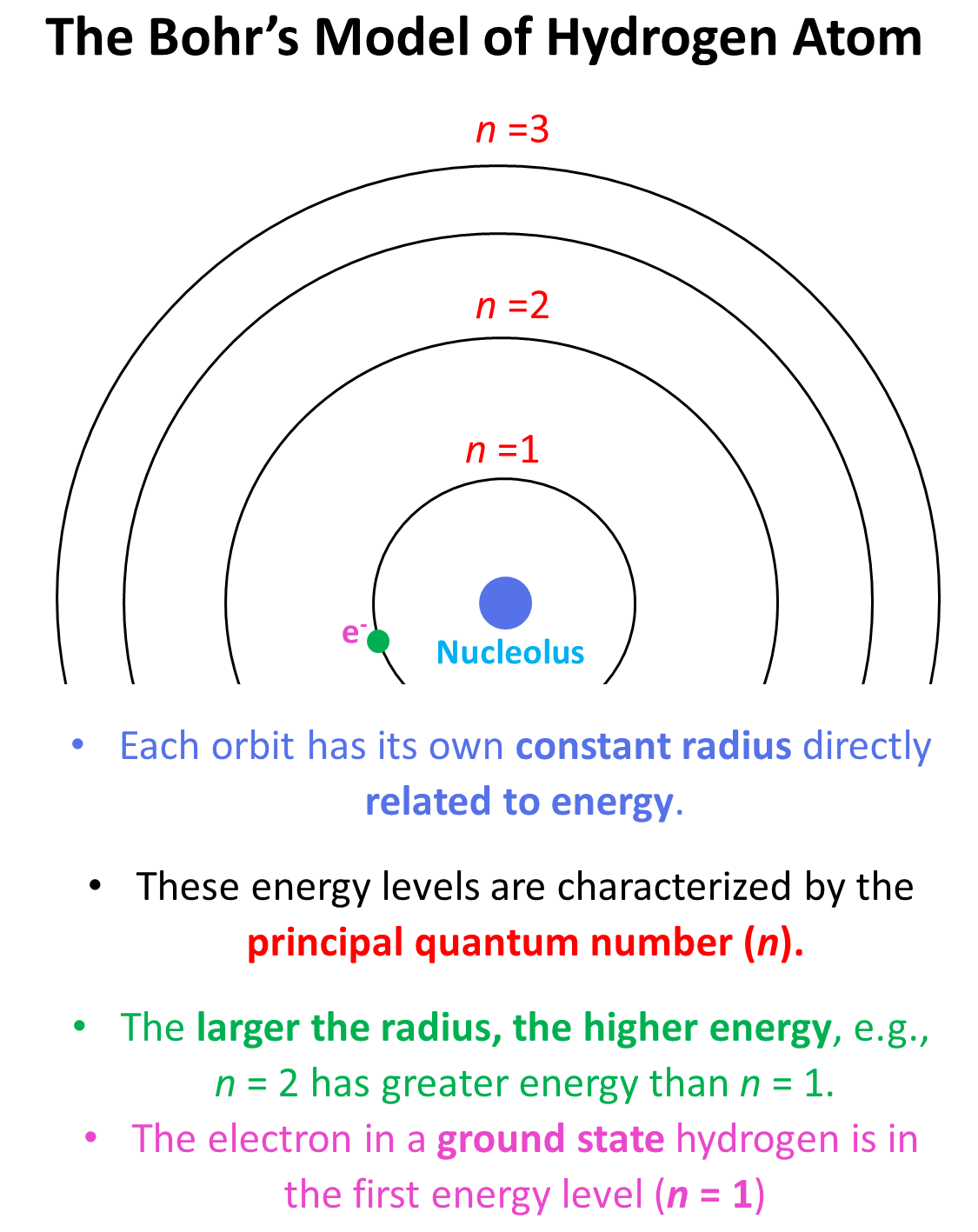The electron in a ground state hydrogen is in the first energy level (n = 1), and it cannot randomly change the orbit without external energy input. On the other hand, once in a higher energy level, it will fall back to the first energy level by losing the same amount of energy as it would need to for the “uphill” transition. In other words, we say that the energy levels of the orbits are quantized and therefore, there are only certain colors of light (photons of certain energy) can be emitted when the electron falls from a higher energy level to a lower energy level.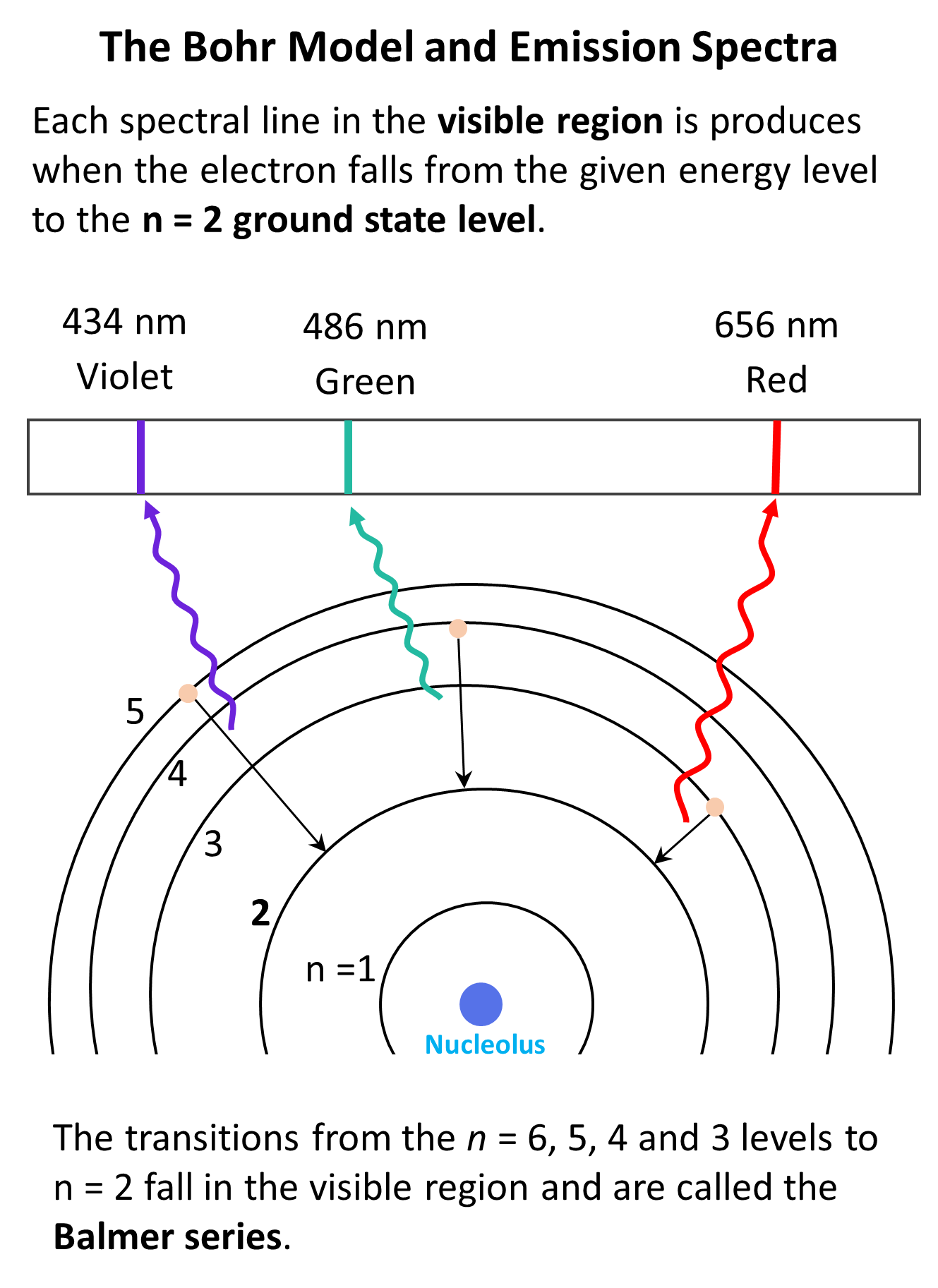The electromagnetic radiation in the visible region emitted from the hydrogen atom corresponds to the transitions of the electron from n = 6, 5, 4, 3 to n = 2 levels. These are called the Balmer series. Notice that the transitions associated with larger n-level gaps correspond to emissions of photos with higher energy. For example, the n = 5 to n = 2 transition emits blue light which is higher in energy (shorter wavelength) than the red light that is emitted when the electron falls from the n = 3 to the n = 2 level.

Now, if the energy difference between the orbits is too large, then the light emitted from the transition won’t fall into the visible region of the spectrum. For example, the transition from n = 6 to n = 1 level corresponds to UV radiation. On the other hand, if the energy is too small then we have infrared (IR) or near IR radiation which we cannot see either.

# Calculating The Wavelength of Electron Transition

The next question we need to address is how to calculate the wavelength of light for electron transitions in the hydrogen atom. For this, Johann Balmer (1825–1898) and Johannes Rydberg (1854–1919) derived a formula correlating the wavelength of emitted photons for the given n levels.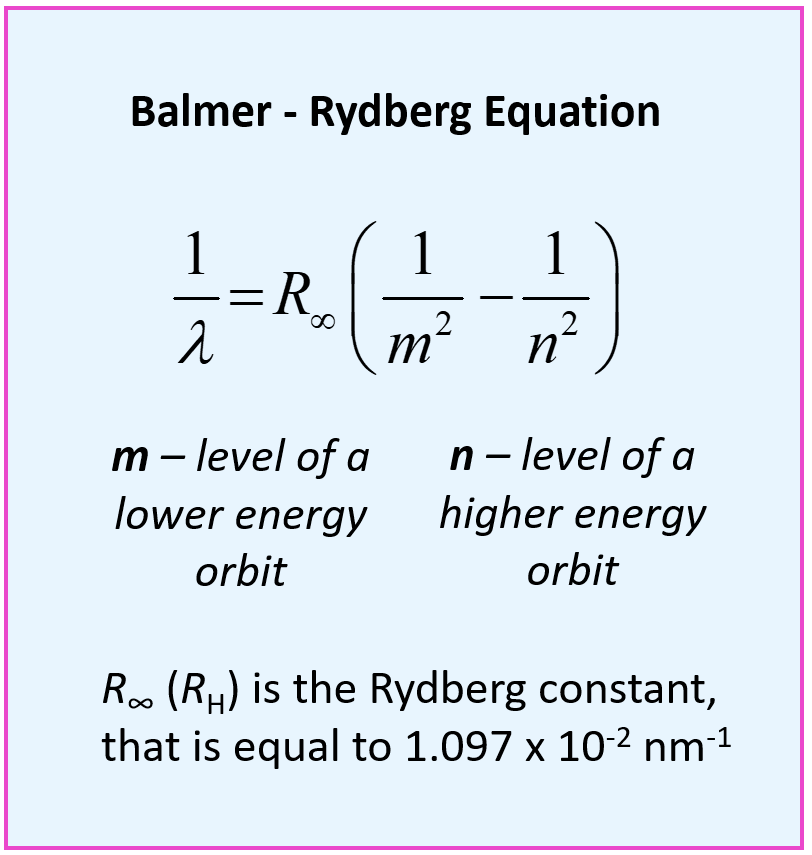This is the Balmer – Rydberg equation where m and n are the lower and higher energy orbits respectively, and R (RH) is the Rydberg constant, which is equal to 1.097 x 10-2 nm-1.

For example, calculate the wavelength of light emitted by a hydrogen atom when an electron moves from = 5 → = 2.

We can first write the Rydberg equation and rearrange it to get an expression for the wavelength:

$\frac{1}{\lambda }\, = \,{R_\infty }\,\left( {\frac{1}{{{m^2}}}\; – \,\frac{1}{{{n^2}}}} \right)$

$\lambda \, = \,\frac{1}{{{R_\infty }\,\left( {\frac{1}{{{m^2}}}\; – \,\frac{1}{{{n^2}}}} \right)}}\,$

And now, we can plug in the numbers:

$\lambda \, = \,\frac{{\rm{1}}}{{{\rm{1}}{\rm{.097}}\,{\rm{ \times }}\,{\rm{1}}{{\rm{0}}^{{\rm{ – 2}}}}{\rm{n}}{{\rm{m}}^{{\rm{ – 1}}}}\,\left( {\frac{{\rm{1}}}{{{{\rm{2}}^{\rm{2}}}}}\;{\rm{ – }}\,\frac{{\rm{1}}}{{{{\rm{5}}^{\rm{2}}}}}} \right)}}\,$

$\lambda \,{\rm{ = }}\,\frac{{\rm{1}}}{{{\rm{1}}{\rm{.097}}\,{\rm{ \times }}\,{\rm{1}}{{\rm{0}}^{{\rm{ – 2}}}}{\rm{n}}{{\rm{m}}^{{\rm{ – 1}}}}\,\left( {\frac{{\rm{1}}}{{\rm{4}}}\;{\rm{ – }}\,\frac{{\rm{1}}}{{{\rm{25}}}}} \right)}}\;{\rm{ = }}\;{\rm{434}}\;{\rm{nm}}\,$

We have seen in the picture above that this is one of the wavelengths in the Balmer series and corresponds to the emission of blue-violet light.

# Calculating The Energy of Electron Transition

The Balmer – Rydberg equation was derived before the Bohr’s model for the hydrogen atom, and Bohr modified it to calculate the values of energy levels and their difference for the electron transitions. He substituted the λ with the corresponding expression based on the correlation of the wavelength and the speed of light which is given as:

$E\; = \,{\rm{ – }}\,hc{R_\infty }\,\left( {\frac{1}{{{n^2}}}} \right)\; = \;{\rm{ – 2}}{\rm{.18}}\, \times \;{\rm{1}}{{\rm{0}}^{{\rm{ – 18}}}}\,{\rm{J}}\,\left( {\frac{1}{{{n^2}}}} \right)$

Where h is the Planck’s constant (6.62 x 10-34 J · s), c is the speed of light (3 x 108 m/s), and RH is the Rydberg constant. Their product gives a new constant expressed in Joules: 2.18 x 10– 18 J.

Notice that there is a negative sign in the formula, and it makes the energies of the electron negative for all values of n. This can be explained by considering that the energy of the electron in the atom is lower than the energy of a free electron.

This is the formula for calculating the energy of a given level. So, how do we calculate the energy of the electron transition of the electron from one orbit (energy level)?  It is calculated according to the difference in the energies of the two orbits:

ΔE = EfinalEinitial = hν

Substituting the frequency (ν) with the corresponding expression for the λ in the Rydberg equation, we obtain a slightly modified Rydberg equation: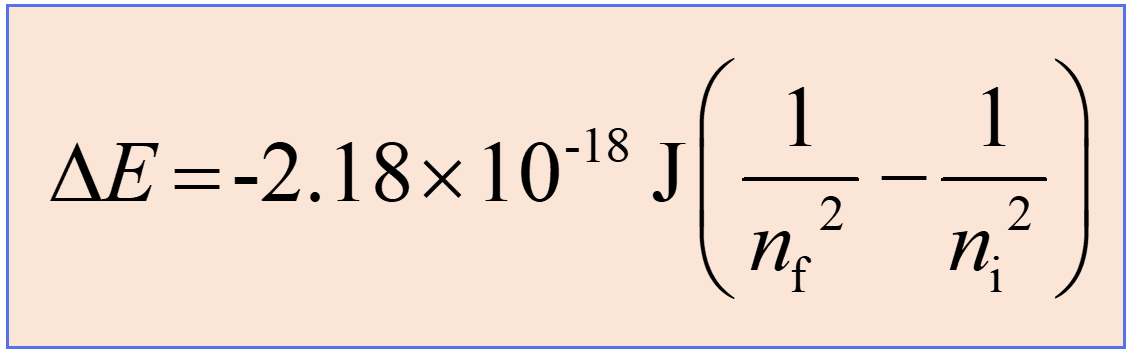Now, if the electron moves from a lower to a higher energy state, then energy is absorbed, and subtracting 1/ni2 from 1/nf2 will allow canceling of the negative signs, thus making ΔE positive. This is what we expect as the energy of the system increases (recall the endothermic and exothermic processes).

On the other hand, when the electron falls from a higher energy state, a photon is released, and the negative sign will stay making ΔE negative meaning the system loses energy. For example, when the electron moves from n = 2 to n = 1, we will have a negative ΔE: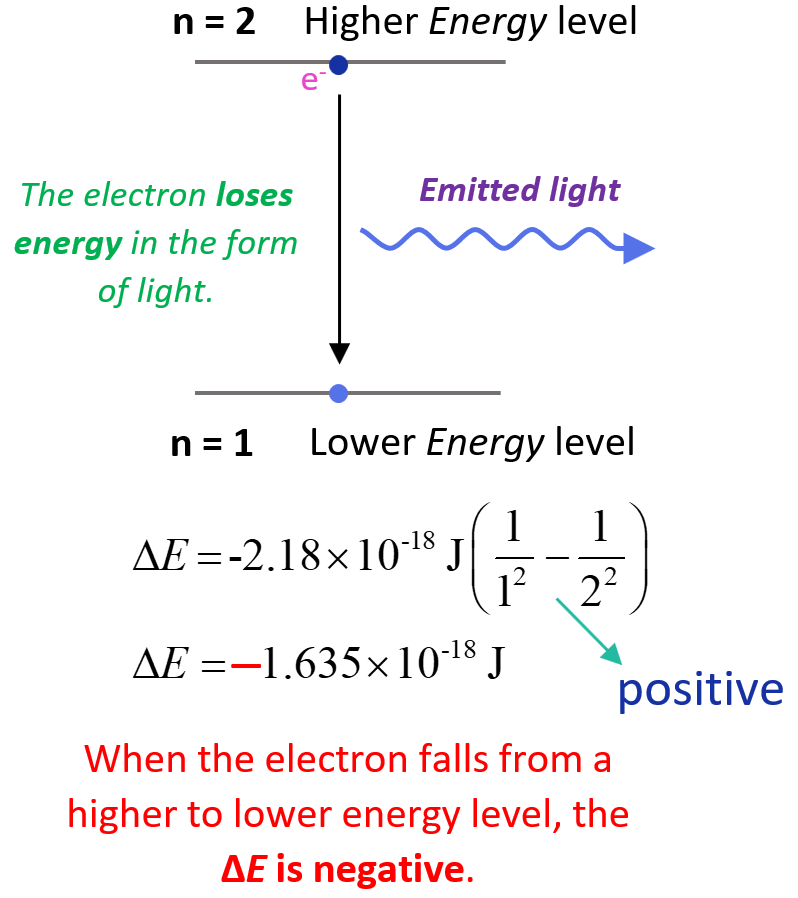The lower (more negative) the energy is, the more stable the atom is. The energy is lowest (most negative) for n = 1. As n gets larger, the energy becomes less negative and therefore increases.

Let’s work on an example of determining the energy of electron transition in the hydrogen atom. Calculate the energy, in Joules, required to excite the electron from n = 1 to n = 3 level in a hydrogen atom.

$\Delta E\, = \,{\rm{ – 2}}{\rm{.18}}\, \times \;{\rm{1}}{{\rm{0}}^{{\rm{ – 18}}}}\,{\rm{J}}\,\left( {\frac{1}{{{n_{\rm{f}}}^2}}\; – \,\frac{1}{{{n_{\rm{i}}}^2}}} \right)$

ni = 1, nf = 3, and therefore, we can write:

${\rm{\Delta E}}\,{\rm{ = }}\,{\rm{ – 2}}{\rm{.18}}\,{\rm{ \times }}\;{\rm{1}}{{\rm{0}}^{{\rm{ – 18}}}}\,{\rm{J}}\,\left( {\frac{{\rm{1}}}{{{{\rm{3}}^{\rm{2}}}}}\;{\rm{ – }}\,\frac{{\rm{1}}}{{{{\rm{1}}^{\rm{2}}}}}} \right)\;{\rm{ = }}\;{\rm{1}}{\rm{.93}}\,{\rm{ \times }}\,{\rm{1}}{{\rm{0}}^{{\rm{ – 18}}}}\,{\rm{J}}$

The energy change is positive and therefore, it is absorbed by the system which is what expect when the electron goes from a lower energy level (in this case ground state as n = 1) to a higher energy level (excited state).

Bohr’s model was a very successful and important contribution to the studies of atomic structure and explaining the line spectrum of hydrogen. In fact, Bohr was awarded the Nobel Prize in Physics in 1922.

However, it is not as applicable to explaining the properties of elements containing more than one electron as the interactions such as electron–electron repulsions must now be accounted for.

Also, as it turned out later, electrons do not move in fixed, defined orbits, and we can never know the precise location of an electron in the atom. This is discussed in more detail in the article about the Heisenberg’s uncertainty principle.

And before moving on to the next topics, here are some more practice examples of the Bohr model of the hydrogen atom that you can work on.

Check Also

#### Practice

1.

Some electron transitions are shown below on a Bohr-model representation of the H atom.(a) Classify each transition as absorption or emission.

(b) Arrange the absorptions in terms of increasing energy.

(c) Rank the emissions in terms of decreasing wavelength of light.

This content is available to registered users only. Click here to Register! By joining Chemistry Steps, you will gain instant access to the answers and solutions for all the Practice Problems and the powerful set of General Chemistry 1 and 2 Summary Study Guides.
Solution
This content is available to registered users only. Click here to Register! By joining Chemistry Steps, you will gain instant access to the answers and solutions for all the Practice Problems and the powerful set of General Chemistry 1 and 2 Summary Study Guides.
2.

For each of the following transitions, use the Rydberg equation to calculate the wavelength of light emitted by a hydrogen atom:

a) n = 3 → n = 2

b) n = 4 → n = 1

c) n = 6 → n = 3

This content is available to registered users only. Click here to Register! By joining Chemistry Steps, you will gain instant access to the answers and solutions for all the Practice Problems and the powerful set of General Chemistry 1 and 2 Summary Study Guides.
Solution
This content is available to registered users only. Click here to Register! By joining Chemistry Steps, you will gain instant access to the answers and solutions for all the Practice Problems and the powerful set of General Chemistry 1 and 2 Summary Study Guides.
3.

What is the maximum wavelength of a photon that can excite the electron from n = 1 to n = 3 level in a hydrogen atom?

This content is available to registered users only. Click here to Register! By joining Chemistry Steps, you will gain instant access to the answers and solutions for all the Practice Problems and the powerful set of General Chemistry 1 and 2 Summary Study Guides.
Solution
This content is available to registered users only. Click here to Register! By joining Chemistry Steps, you will gain instant access to the answers and solutions for all the Practice Problems and the powerful set of General Chemistry 1 and 2 Summary Study Guides.
4.

What is the energy level that the electron moves when a hydrogen atom at ground state absorbs a photon of wavelength 95 nm?

This content is available to registered users only. Click here to Register! By joining Chemistry Steps, you will gain instant access to the answers and solutions for all the Practice Problems and the powerful set of General Chemistry 1 and 2 Summary Study Guides.
Solution
This content is available to registered users only. Click here to Register! By joining Chemistry Steps, you will gain instant access to the answers and solutions for all the Practice Problems and the powerful set of General Chemistry 1 and 2 Summary Study Guides.
5.

Would a photon of 115 nm light have sufficient energy to excite an electron in a ground state hydrogen atom to the n = 4 energy state?